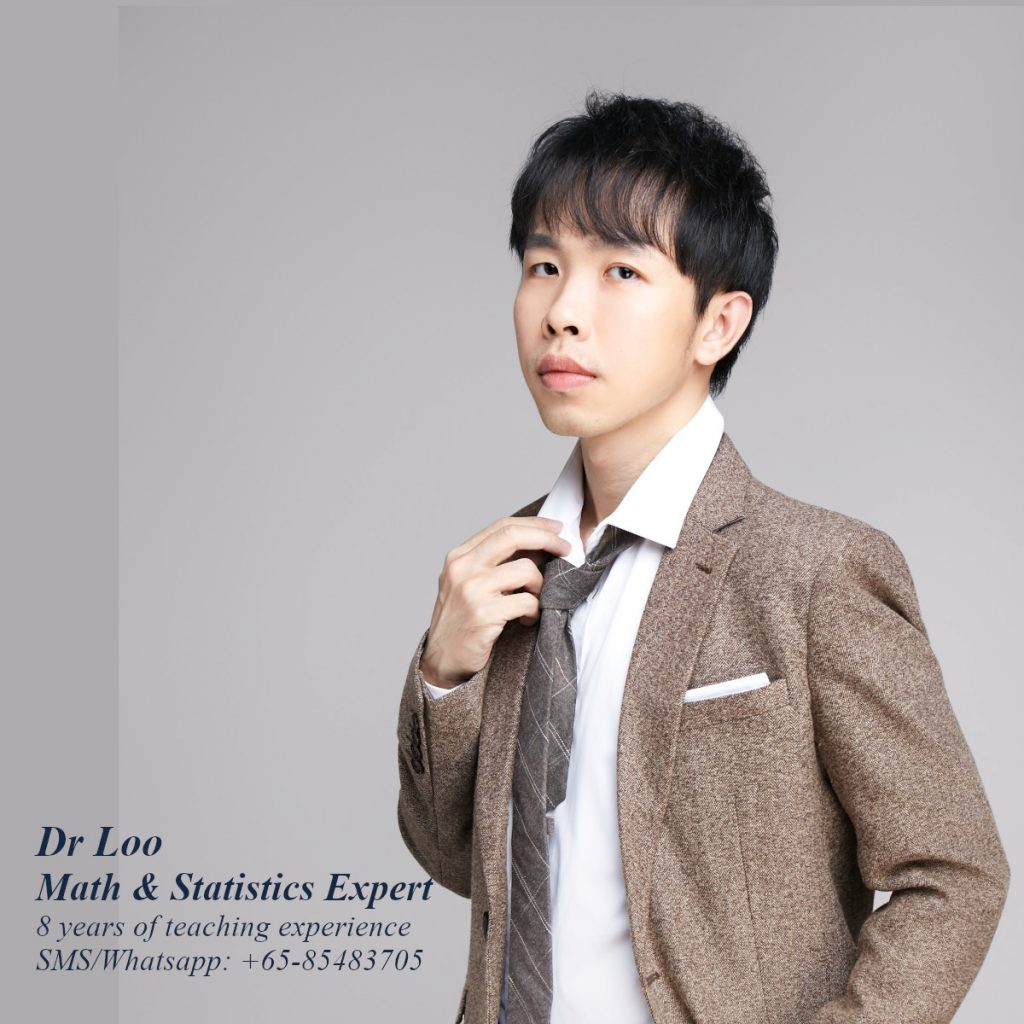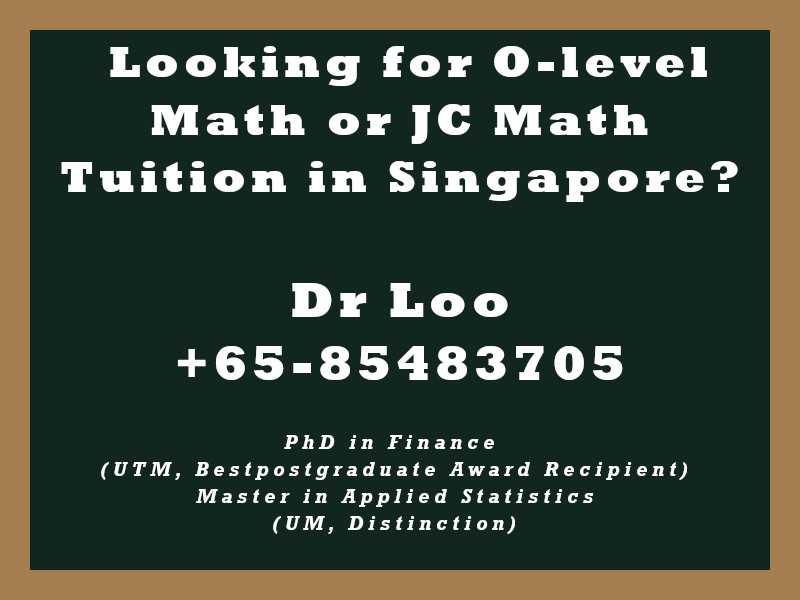+65-85483705
Select Page

# Centre and Radius of a circle

Question

From the of the circles equations, determine the radius and also the centre.

1. 32 = x2 + y2
2. -30 = x2 + y2 – 6x – 10y

1. Radius = 3, Centre = (0,0)
2. Radius = 2, Centre = (3,5)
Note:

The equation of a circle
(x-h)2 + (y-b)2 = r2
Where
Center = (h,b)

General form of circle equation
ax2 + by2 + cx + dy + e = 0

From General form of circle equation to The equation of a circle:
Perform completing the square on the two variables in the equation.

## About the Math Tutor - Dr Loo

I am a PhD holder with 9 years of teaching experience at secondary school and university. My expertizes are mathematics, statistics, econometrics, finance and machine learning.

Currently I do provide consultation and private tuition in Singapore. For those who are looking for math tutor in Singapore or statistics tutor in Singapore, please feel free to contact me at +65-85483705 (SMS/Whatsapp/Telegram).

Some of the math tuition in Singapore I provide includes O-level math tuition and also JC Math Tuition (H1 Math Tuition & H2 Math Tuition). On the other hand, my statistics tuition in Singapore mostly focus on pre-university level until postgraduate level. For those who prefer online tutoring service, the online O-level math tuition and online JC math tuition are also available.## For those who are looking for math tution in Singapore

Need help with this topic? I do provide mathematics home tuition in Singapore for O-level math and also JC H2 math. In addition, online math tutoring is available as well. Feel free to contact me if you would like to know further.